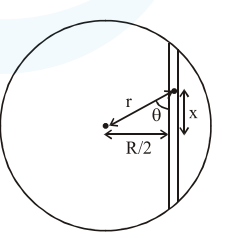Deepak Scored 45->99%ile with Bounce Back Crack Course. You can do it too!

# Assume that a tunnel is dug along a chord of the earth, at a perpendicular distance

Question:

Assume that a tunnel is dug along a chord of the earth, at a perpendicular distance $(\mathrm{R} / 2)$ from the earth's centre, where ' $R$ ' is the radius of the Earth. The wall of the tunnel is frictionless. If a particle is released in this tunnel, it will execute a simple harmonic motion with a time period:

1. $\frac{2 \pi R}{g}$

2. $\frac{g}{2 \pi R}$

3. $\frac{1}{2 \pi} \sqrt{\frac{\mathrm{g}}{\mathrm{R}}}$

4. $2 \pi \sqrt{\frac{\mathrm{R}}{\mathrm{g}}}$

Correct Option: , 4

Solution:Force along the tunnel

$\mathrm{F}=-\left(\frac{\mathrm{GMmr}}{\mathrm{R}^{3}}\right) \cos \theta$

$\mathrm{F}=-\frac{\mathrm{gm}}{\mathrm{R}} \mathrm{x}\left(\frac{\mathrm{GM}}{\mathrm{R}^{2}}=\mathrm{g}, \mathrm{r} \cos \theta=\mathrm{x}\right)$

$a=-\frac{g}{R} x$

$\omega^{2}=\frac{g}{R} \quad T=2 \pi \sqrt{\frac{R}{g}}$